# Recognizers 03 Mar21 Parsers and recognizers n Given

• Slides: 32
Download presentationRecognizers 03 -Mar-21Parsers and recognizers n Given a grammar (say, in BNF) and a string, n n A recognizer will tell whether the string belongs to the language defined by the grammar A parser will try to build a tree corresponding to the string, according to the rules of the grammar Input string Recognizer result 2 + 3 * 4 true 2 + 3 * false Parser result Error 2Building a recognizer n n One way of building a recognizer from a grammar is called recursive descent Recursive descent is pretty easy to implement, once you figure out the basic ideas n n n Recursive descent is a great way to build a “quick and dirty” recognizer or parser Production-quality parsers use much more sophisticated and efficient techniques In the following slides, I’ll talk about how to do recursive descent, and give some examples in Java 3Review of BNF and EBNF n n “Plain” BNF n < > indicate a nonterminal that needs to be further expanded, for example, <variable> n Symbols not enclosed in < > are terminals; they represent themselves, for example, if, while, ( n The symbol : : = means is defined as n The symbol | means or; it separates alternatives, for example, <addop> : : = + | Extended BNF n [ ] enclose an optional part of the rule n n Example: <if statement> : : = if ( <condition> ) <statement> [ else <statement> ] { } mean the enclosed can be repeated zero or more times n Example: <parameter list> : : = ( ) | ( { <parameter> , } <parameter> ) 4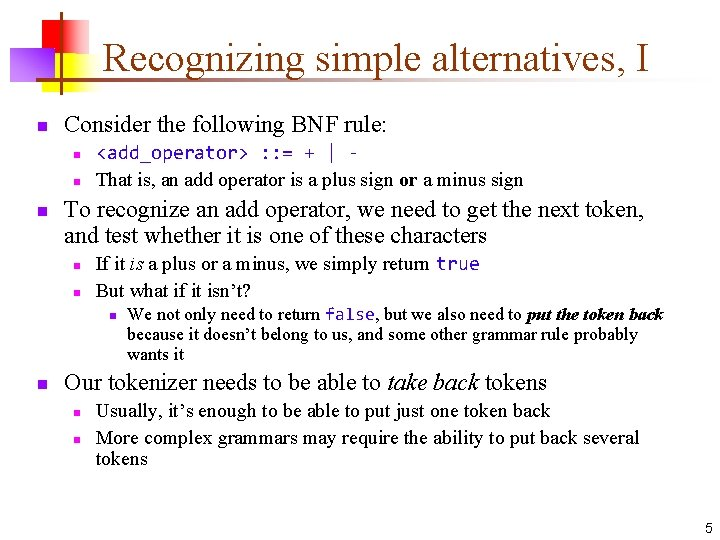Recognizing simple alternatives, I n Consider the following BNF rule: n n n <add_operator> : : = + | That is, an add operator is a plus sign or a minus sign To recognize an add operator, we need to get the next token, and test whether it is one of these characters n n If it is a plus or a minus, we simply return true But what if it isn’t? n n We not only need to return false, but we also need to put the token back because it doesn’t belong to us, and some other grammar rule probably wants it Our tokenizer needs to be able to take back tokens n n Usually, it’s enough to be able to put just one token back More complex grammars may require the ability to put back several tokens 5Recognizing simple alternatives, II n n Our rule is <add_operator> : : = + | Our method for recognizing an <add_operator> (which we will call is. Add. Operator) looks like this: n public boolean is. Add. Operator() { Get the next token, call it t If t is a “+”, return true If t is a “-”, return true If t is anything else, put the token back return false } 6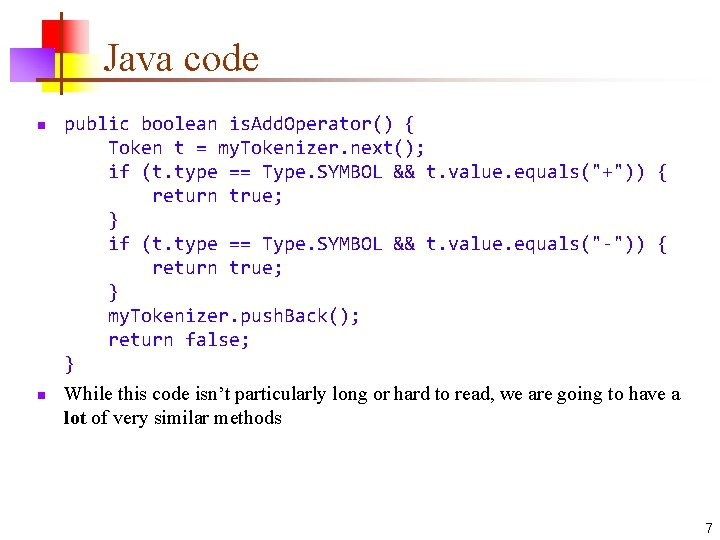Java code n n public boolean is. Add. Operator() { Token t = my. Tokenizer. next(); if (t. type == Type. SYMBOL && t. value. equals("+")) { return true; } if (t. type == Type. SYMBOL && t. value. equals("-")) { return true; } my. Tokenizer. push. Back(); return false; } While this code isn’t particularly long or hard to read, we are going to have a lot of very similar methods 7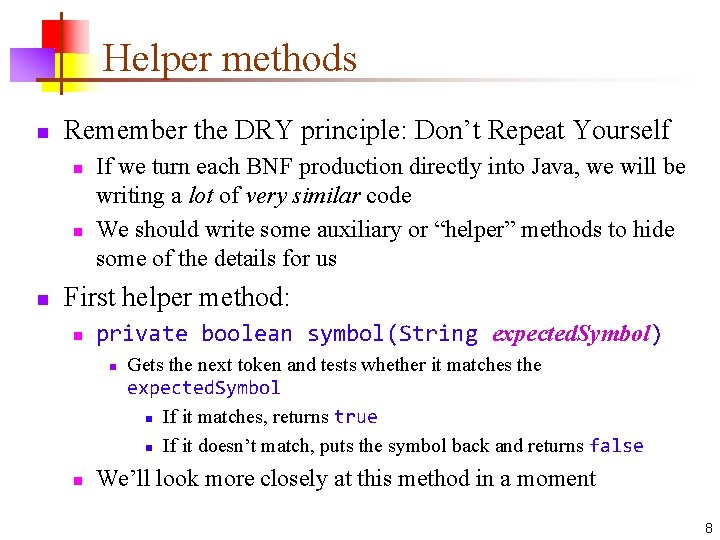Helper methods n Remember the DRY principle: Don’t Repeat Yourself n n n If we turn each BNF production directly into Java, we will be writing a lot of very similar code We should write some auxiliary or “helper” methods to hide some of the details for us First helper method: n private boolean symbol(String expected. Symbol) n n Gets the next token and tests whether it matches the expected. Symbol n If it matches, returns true n If it doesn’t match, puts the symbol back and returns false We’ll look more closely at this method in a moment 8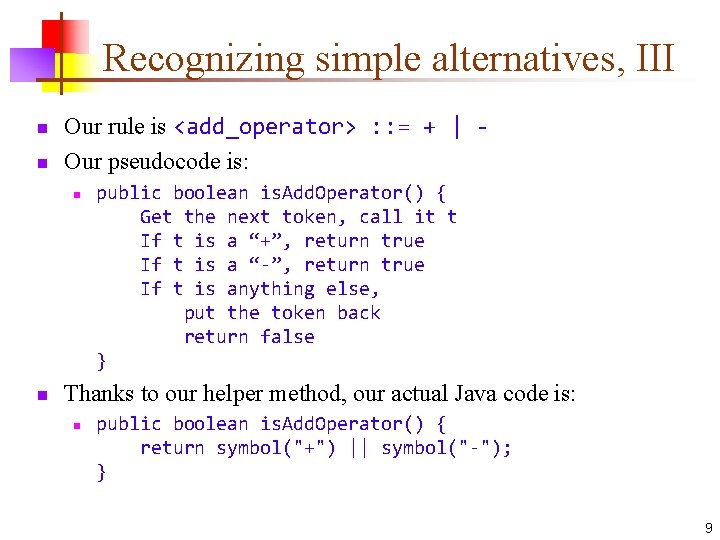Recognizing simple alternatives, III n n Our rule is <add_operator> : : = + | Our pseudocode is: n n public boolean is. Add. Operator() { Get the next token, call it t If t is a “+”, return true If t is a “-”, return true If t is anything else, put the token back return false } Thanks to our helper method, our actual Java code is: n public boolean is. Add. Operator() { return symbol("+") || symbol("-"); } 9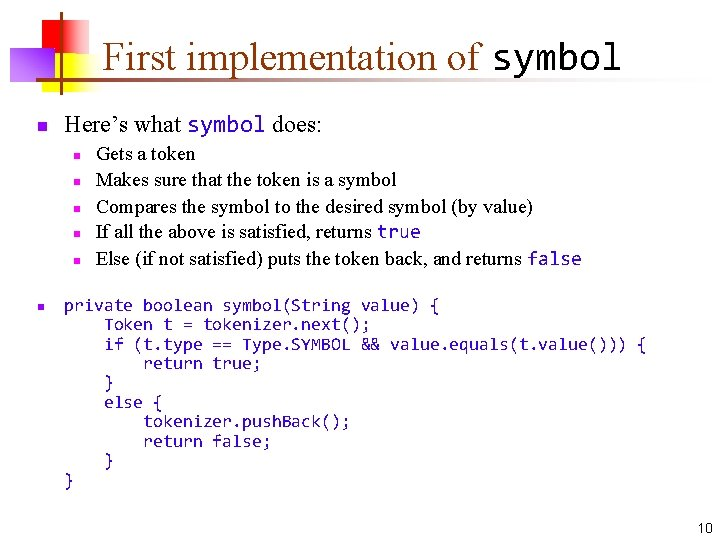First implementation of symbol n Here’s what symbol does: n n n Gets a token Makes sure that the token is a symbol Compares the symbol to the desired symbol (by value) If all the above is satisfied, returns true Else (if not satisfied) puts the token back, and returns false private boolean symbol(String value) { Token t = tokenizer. next(); if (t. type == Type. SYMBOL && value. equals(t. value())) { return true; } else { tokenizer. push. Back(); return false; } } 10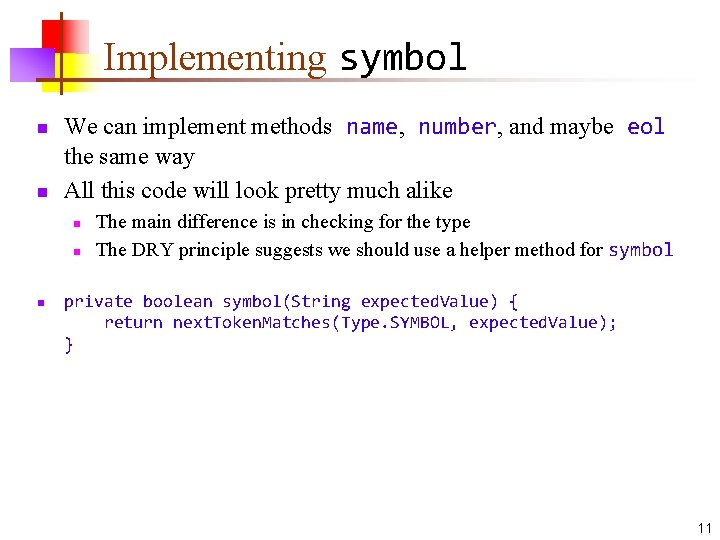Implementing symbol n n We can implement methods name, number, and maybe eol the same way All this code will look pretty much alike n n n The main difference is in checking for the type The DRY principle suggests we should use a helper method for symbol private boolean symbol(String expected. Value) { return next. Token. Matches(Type. SYMBOL, expected. Value); } 11next. Token. Matches #1 n The next. Token. Matches method should: n n n Get a token Compare types and values Return true if the token is as expected Put the token back and return false if it doesn’t match private boolean next. Token. Matches(Type type, String value) { Token t = tokenizer. next(); if (type == t. type() && value. equals(t. value())) { return true; } else { tokenizer. push. Back(1); return false; } } 12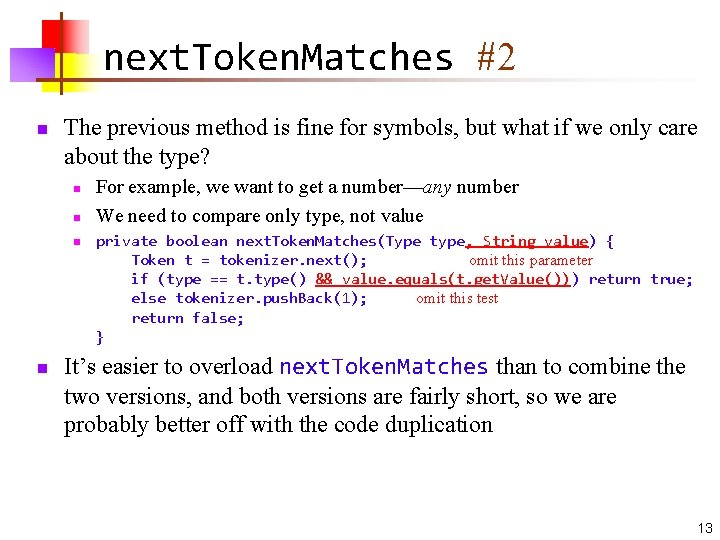next. Token. Matches #2 n The previous method is fine for symbols, but what if we only care about the type? n n For example, we want to get a number—any number We need to compare only type, not value private boolean next. Token. Matches(Type type, String value) { Token t = tokenizer. next(); omit this parameter if (type == t. type() && value. equals(t. get. Value())) return true; else tokenizer. push. Back(1); omit this test return false; } It’s easier to overload next. Token. Matches than to combine the two versions, and both versions are fairly short, so we are probably better off with the code duplication 13add. Operator reprise n n n public boolean is. Add. Operator() { return symbol("+") || symbol("-"); } private boolean symbol(String expected. Value) { return next. Token. Matches(Type. SYMBOL, expected. Value); } private boolean next. Token. Matches(Type type, String value) { Token t = tokenizer. next(); if (type == t. type() && value. equals(t. value())) return true; else tokenizer. push. Back(); return false; } 14Sequences, I n Suppose we want to recognize a grammar rule in which one thing follows another, for example, n n n <empty_list> : : = “[” “]” (I put quotes around these brackets to distinguish them from the EBNF metasymbols for “optional”) Here’s some code we might try: n public boolean is. Empty. List() { return symbol("[") && symbol("]"); } 15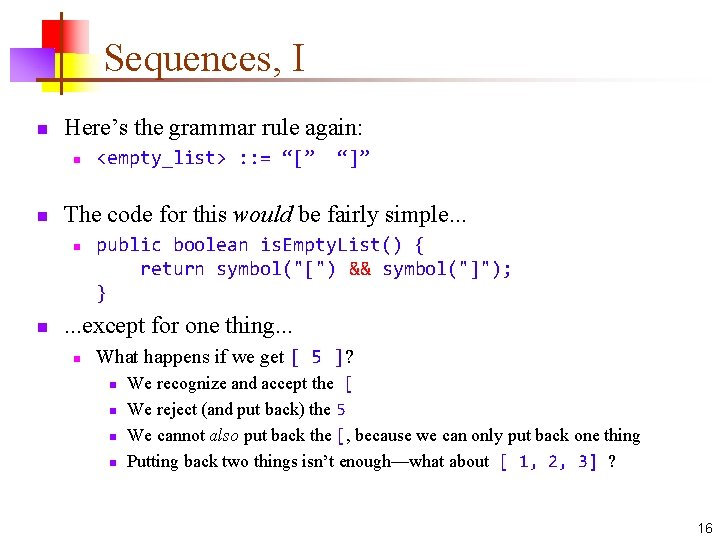Sequences, I n Here’s the grammar rule again: n n “]” The code for this would be fairly simple. . . n n <empty_list> : : = “[” public boolean is. Empty. List() { return symbol("[") && symbol("]"); } . . . except for one thing. . . n What happens if we get [ 5 ]? n n We recognize and accept the [ We reject (and put back) the 5 We cannot also put back the [, because we can only put back one thing Putting back two things isn’t enough—what about [ 1, 2, 3] ? 16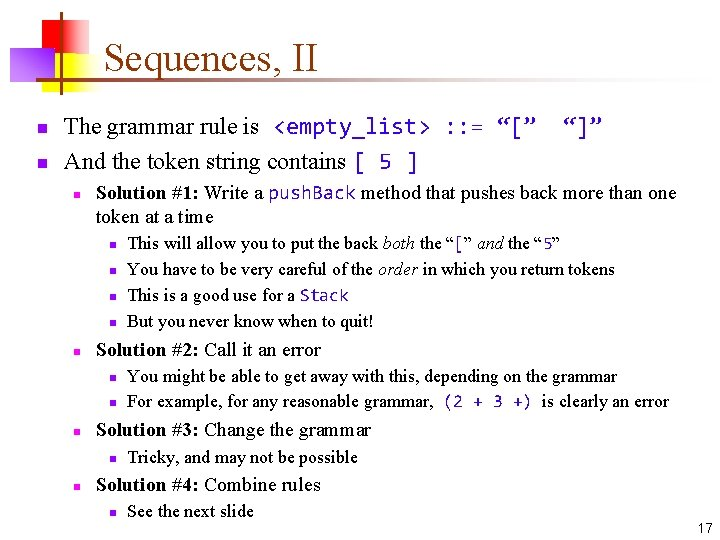Sequences, II n n The grammar rule is <empty_list> : : = “[” And the token string contains [ 5 ] n Solution #1: Write a push. Back method that pushes back more than one token at a time n n n You might be able to get away with this, depending on the grammar For example, for any reasonable grammar, (2 + 3 +) is clearly an error Solution #3: Change the grammar n n This will allow you to put the back both the “[” and the “ 5” You have to be very careful of the order in which you return tokens This is a good use for a Stack But you never know when to quit! Solution #2: Call it an error n n “]” Tricky, and may not be possible Solution #4: Combine rules n See the next slide 17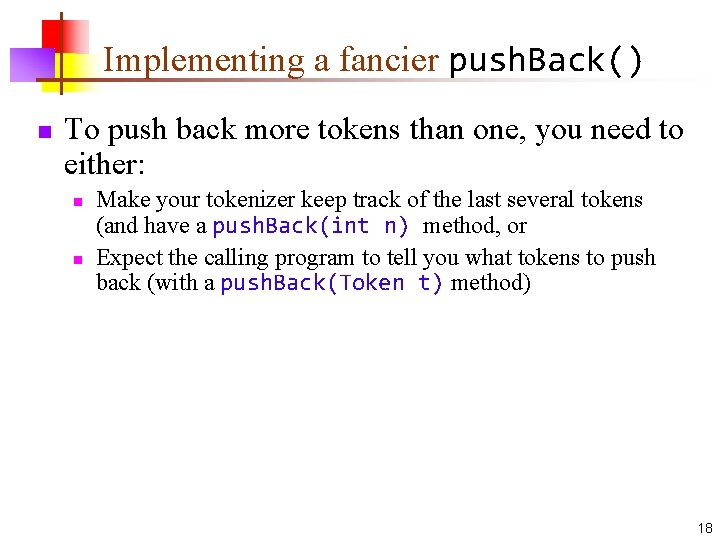Implementing a fancier push. Back() n To push back more tokens than one, you need to either: n n Make your tokenizer keep track of the last several tokens (and have a push. Back(int n) method, or Expect the calling program to tell you what tokens to push back (with a push. Back(Token t) method) 18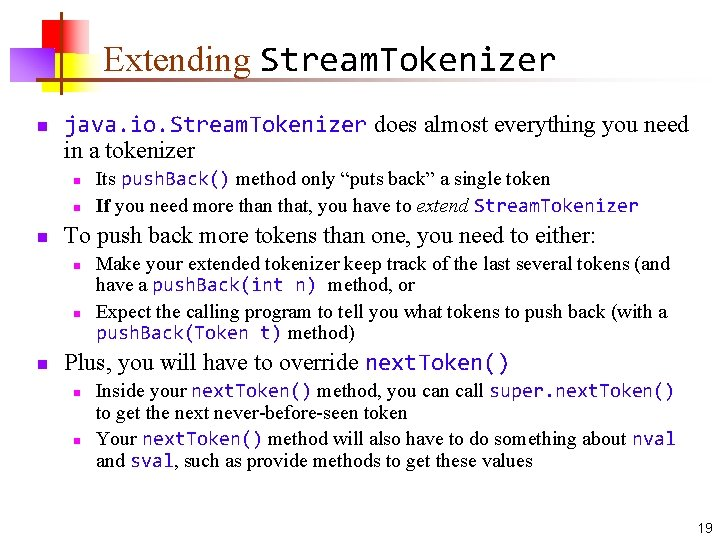Extending Stream. Tokenizer n java. io. Stream. Tokenizer does almost everything you need in a tokenizer n n n To push back more tokens than one, you need to either: n n n Its push. Back() method only “puts back” a single token If you need more than that, you have to extend Stream. Tokenizer Make your extended tokenizer keep track of the last several tokens (and have a push. Back(int n) method, or Expect the calling program to tell you what tokens to push back (with a push. Back(Token t) method) Plus, you will have to override next. Token() n n Inside your next. Token() method, you can call super. next. Token() to get the next never-before-seen token Your next. Token() method will also have to do something about nval and sval, such as provide methods to get these values 19Sequences, III n n Suppose the grammar really says <list> : : = “[” “]” | “[” <number> “]” Now your pseudocode should look something like this: n public boolean list() { if first token is “[” { if second token is “]” return true else if second token is a number { if third token is “]” return true else error } else put back first token } 20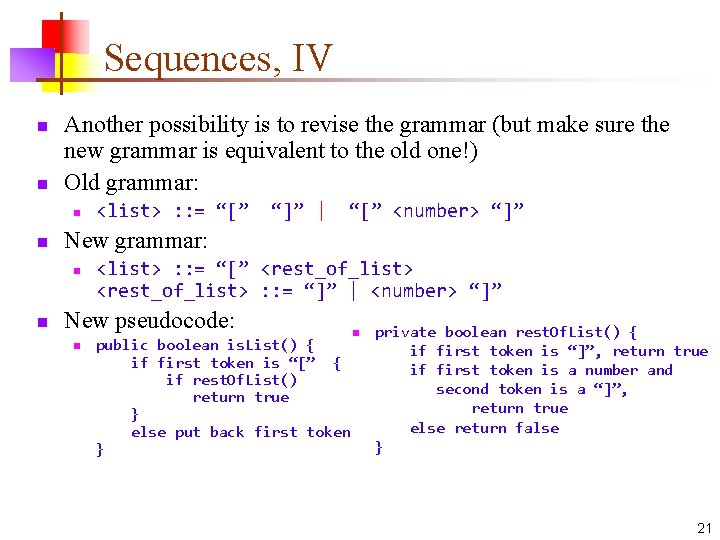Sequences, IV n n Another possibility is to revise the grammar (but make sure the new grammar is equivalent to the old one!) Old grammar: n n “]” | “[” <number> “]” New grammar: n n <list> : : = “[” <rest_of_list> : : = “]” | <number> “]” New pseudocode: n public boolean is. List() { if first token is “[” { if rest. Of. List() return true } else put back first token } n private boolean rest. Of. List() { if first token is “]”, return true if first token is a number and second token is a “]”, return true else return false } 21Simple sequences in Java n Suppose you have this rule: n n n <factor> : : = ( <expression> ) A good way to do this is often to test whether the grammar rule is not met public boolean is. Factor() { if (symbol("(")) { if (!is. Expression()) { error("Error in parenthesized expression"); } if (!symbol(")")) { error("Unclosed parenthetical expression"); } return true; } return false; } To do this, you need to be careful that the “(” is not the start of some other production that can be used where a factor can be used n In other words, be sure that if you get a “(” it must start a factor Also, error(String) must throw an Exception—why? 22false vs. error n When should a method return false, and when should it report an error? n n false means that this method did not recognize its input Report an error if you know that something has gone wrong n n In other words, you know that no other method will recognize the input, either public boolean is. If. Statement() { if you don’t see “if”, return false // could be some other kind of statement if you don’t see a condition, return an error // “if” is a keyword that must start an if statement If you see if, and it isn’t followed by a condition, there is nothing else that it could be This isn’t completely mechanical; you have to decide 23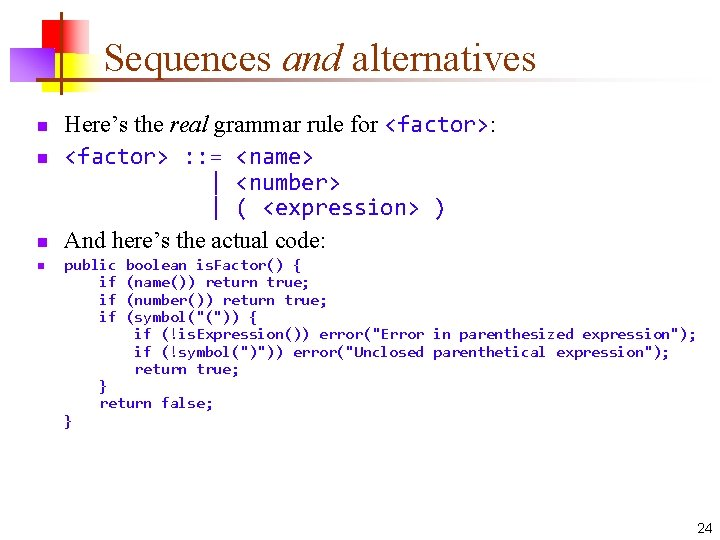Sequences and alternatives n n Here’s the real grammar rule for <factor>: <factor> : : = <name> | <number> | ( <expression> ) And here’s the actual code: public if if if } boolean is. Factor() { (name()) return true; (number()) return true; (symbol("(")) { if (!is. Expression()) error("Error in parenthesized expression"); if (!symbol(")")) error("Unclosed parenthetical expression"); return true; } return false; 24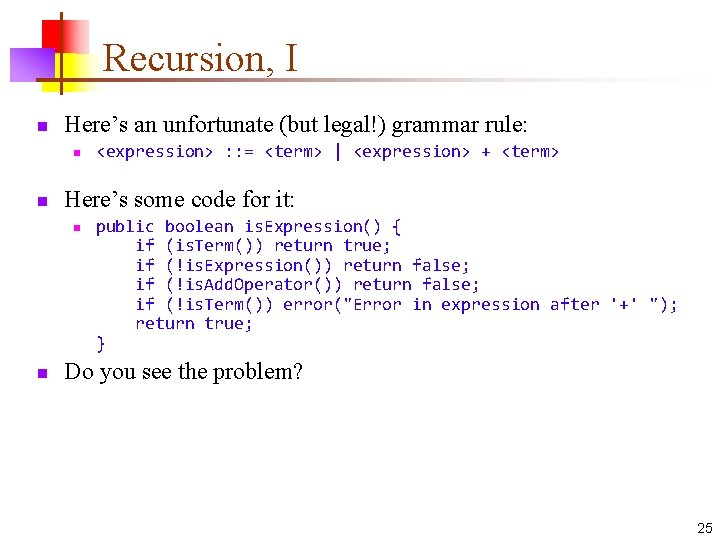Recursion, I n Here’s an unfortunate (but legal!) grammar rule: n n Here’s some code for it: n n <expression> : : = <term> | <expression> + <term> public boolean is. Expression() { if (is. Term()) return true; if (!is. Expression()) return false; if (!is. Add. Operator()) return false; if (!is. Term()) error("Error in expression after '+' "); return true; } Do you see the problem? 25Recursion, I n Here’s the rule again: n n And the code: n n n <expression> : : = <term> | <expression> + <term> public boolean is. Expression() { if (is. Term()) return true; if (!is. Expression()) return false; if (!is. Add. Operator()) return false; if (!is. Term()) error("Error in expression after '+' "); return true; } We aren’t recurring with a simpler case, therefore, we have an infinite recursion Our grammar rule is left recursive (the recursive part is the leftmost thing in the definition) 26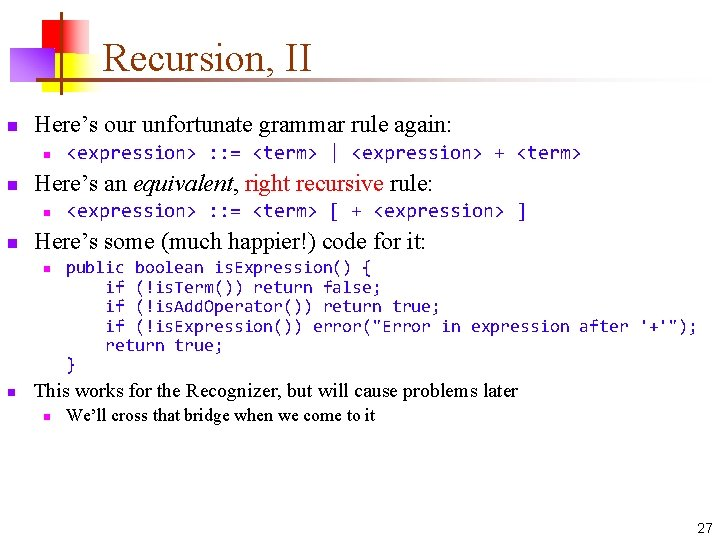Recursion, II n Here’s our unfortunate grammar rule again: n n Here’s an equivalent, right recursive rule: n n <expression> : : = <term> [ + <expression> ] Here’s some (much happier!) code for it: n n <expression> : : = <term> | <expression> + <term> public boolean is. Expression() { if (!is. Term()) return false; if (!is. Add. Operator()) return true; if (!is. Expression()) error("Error in expression after '+'"); return true; } This works for the Recognizer, but will cause problems later n We’ll cross that bridge when we come to it 27Extended BNF—optional parts n Extended BNF uses brackets to indicate optional parts of rules n n Example: <if_statement> : : = if <condition> <statement> [ else <statement> ] Pseudocode for this example: public boolean is. If. Statement() { if you don’t see “if”, return false if you don’t see a condition, return an error if you don’t see a statement, return an error if you see an “else” { if you see a “statement”, return true else return an error } else return true; } 28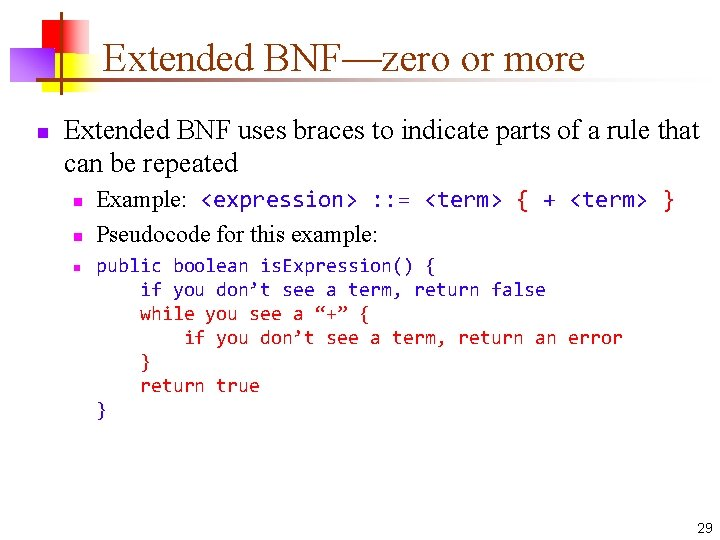Extended BNF—zero or more n Extended BNF uses braces to indicate parts of a rule that can be repeated n n n Example: <expression> : : = <term> { + <term> } Pseudocode for this example: public boolean is. Expression() { if you don’t see a term, return false while you see a “+” { if you don’t see a term, return an error } return true } 29Back to parsers n n A parser is like a recognizer The difference is that, when a parser recognizes something, it does something about it n n Usually, what a parser does is build a tree If the thing that is being parsed is a program, then n You can write another program that “walks” the tree and executes the statements and expressions as it finds them n n Such a program is called an interpreter You can write another program that “walks” the tree and produces code in some other language (usually assembly language) that does the same thing n Such a program is called a compiler 30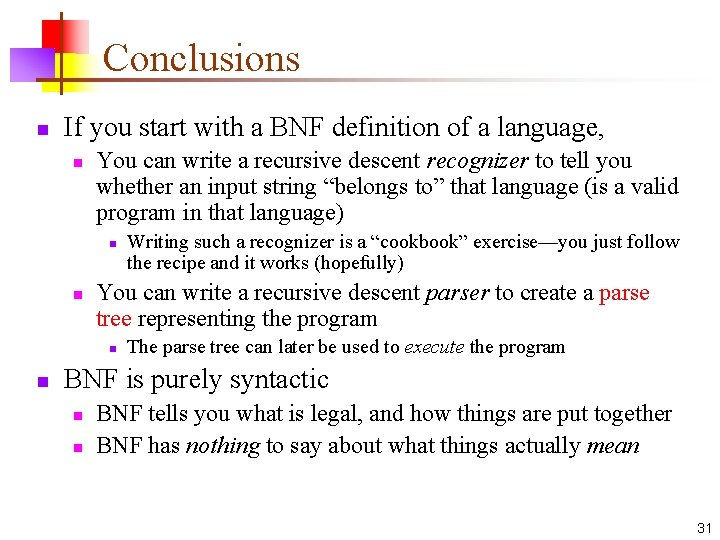Conclusions n If you start with a BNF definition of a language, n You can write a recursive descent recognizer to tell you whether an input string “belongs to” that language (is a valid program in that language) n n You can write a recursive descent parser to create a parse tree representing the program n n Writing such a recognizer is a “cookbook” exercise—you just follow the recipe and it works (hopefully) The parse tree can later be used to execute the program BNF is purely syntactic n n BNF tells you what is legal, and how things are put together BNF has nothing to say about what things actually mean 31The End 32